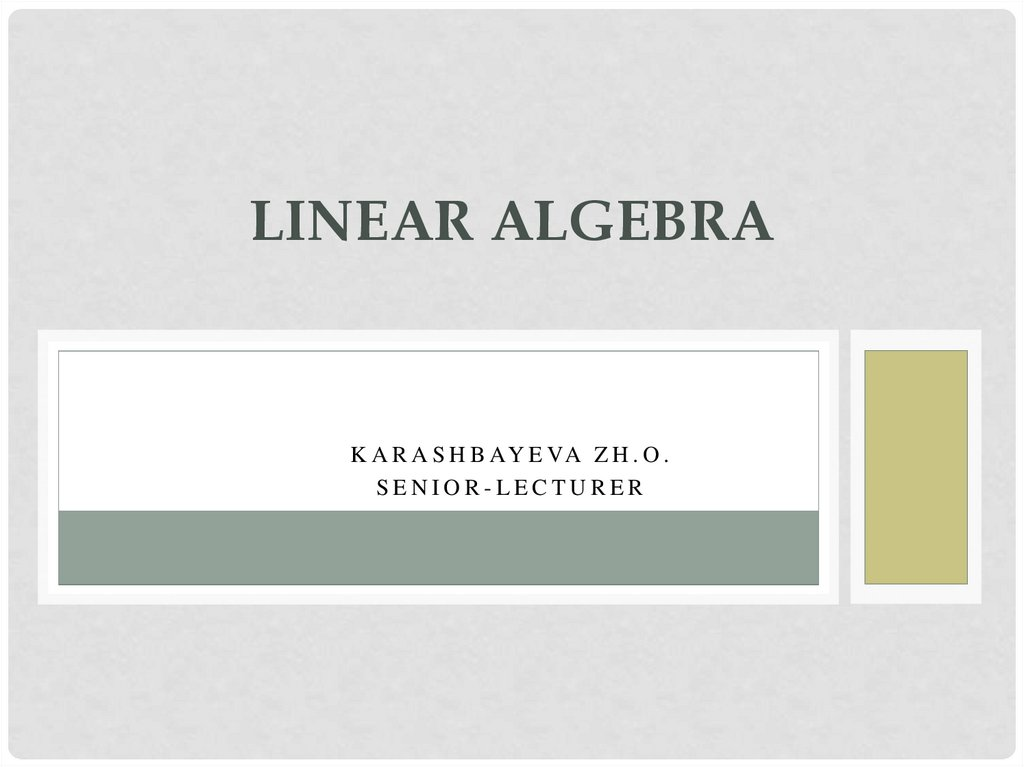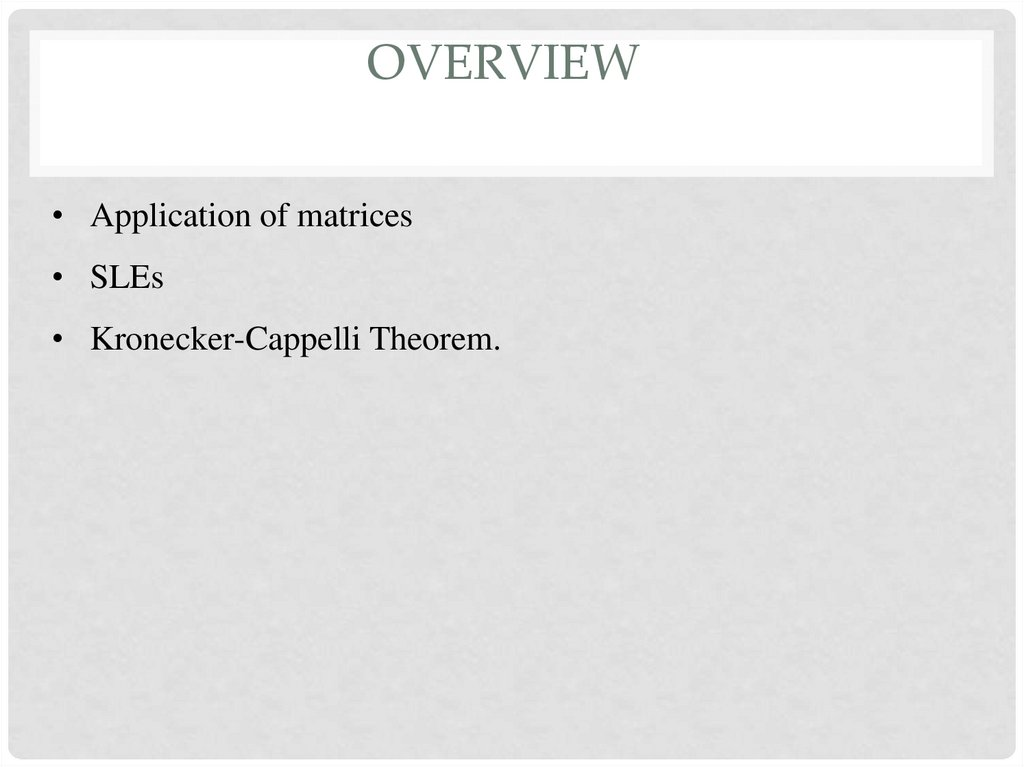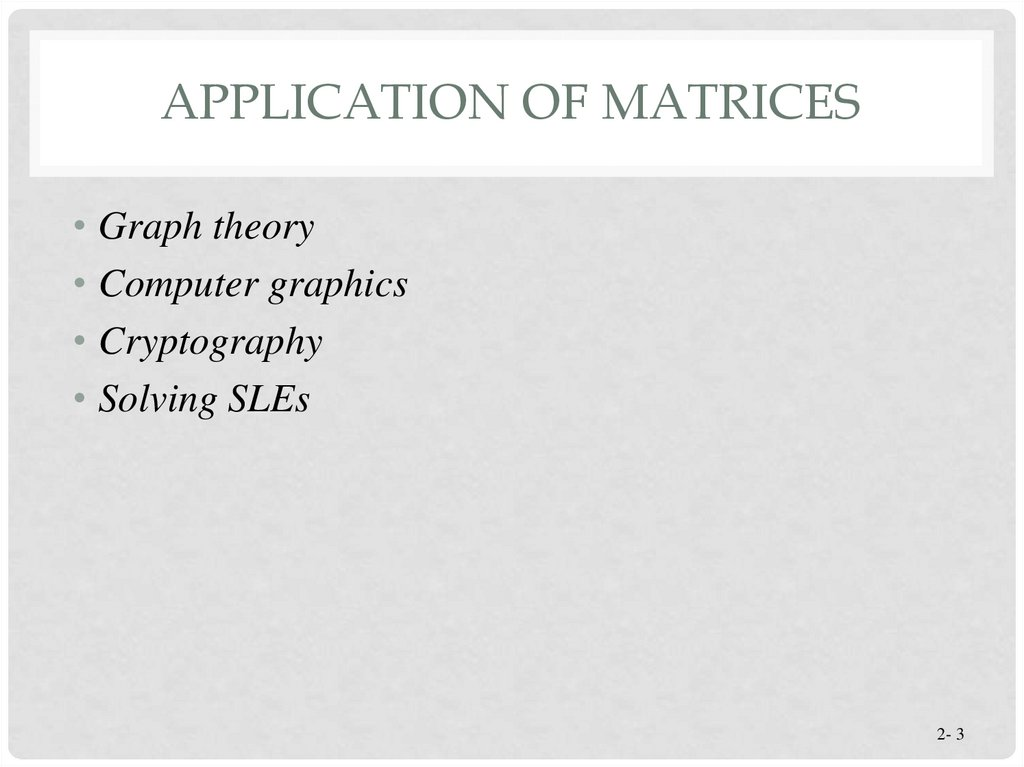# Linear Algebra. Lecture 5

## 1. Linear Algebra

LINEAR ALGEBRA
K A R A S H B AY E VA Z H . O .
SENIOR-LECTURER

## 2. overview

OVERVIEW
• Application of matrices
• SLEs
• Kronecker-Cappelli Theorem.

## 3. Application of matrices

APPLICATION OF MATRICES
• Graph theory
• Computer graphics
• Cryptography
• Solving SLEs
2- 3

GRAPH THEORY

## 5. Computer graphics

COMPUTER GRAPHICS

## 6. Computer graphics

COMPUTER GRAPHICS

## 7. Computer graphics

COMPUTER GRAPHICS

## 8. Cryptography

CRYPTOGRAPHY
• Study of encoding and decoding
secret messages
• Useful in sending sensitive information
so that only the intended receivers
can understand the message
• A common use of cryptography is to
send government secrets.

## 9. Encoding

A1
B2
C3
D4
E5
F6
G7
H8
I9
J 10
K 11
L 12
M 13
N 14
O 15
P 16
Q 17
ENCODING
R 18
S 19
• First we will assign numbers to
represent each letter of the alphabet. T 20
U 21
• We then create a “plaintext” matrix V 22
that holds the message in terms of
W 23
numbers.
X 24
Y 25
• Then we pick an invertible square
matrix, which can be multiplied with Z 26
_ 27
the “plaintext matrix”.
‘ 28

## 10.

1 2 1
2
2
4
1 3 3
Encrypting the Message
x
6 18 5 5 27 12 1 21 14 4 18 25 27
13 15 14 5 25 27 21 14 4 5 18 27 19
15 13 5 15 14 5 28 19 27 4 5 19 11
Encoding matrix
=
Plaintext
17 35 28 0
63 61 15 30 5 10 49 60 54
98 118 58 80 160 98 156 146 144 34 92 180 136
0 24 32 25 60 78 20 6 55 7 57 49 51
Ciphertext

## 11. Deciphering the Message

DECIPHERING THE MESSAGE
• In order to decode the message, we would have to take
the inverse of the encoding matrix to obtain the decoding
matrix.
• Multiplying the decoding matrix with the ciphertext would
result in the plaintext version.
• Then the arbitrarily assigned number scheme can be used
to retrieve the message.

## 12.

A1
B2
C3
D4
E5
F6
G7
H8
I9
J 10
K 11
L 12
M 13
N 14
Decrypting the Message
9 3
5
2
3
5 1
2 1
2 1
x
17 35 28 0
63 61 15 30 5 10 49 60
98 118 58 80 160 98 156 146 144 34 92 180
0 24 32 25 60 78 20 6 55 7 57 49
=
6 18 5 5 27 12 1 21 14 4 18 25
13 15 14 5 25 27 21 14 4 5 18 27
15 13 5 15 14 5 28 19 27 4 5 19
O 15
P 16
Q 17
R 18
S 19
T 20
U 21
54
V 22
136
W 23
51
X 24
Y 25
27
Z 26
19 _ 27
11
‘ 28

## 13.

YOU MIGHT WANT TO READ THIS.
A1
B2
C3
D4
E5
F6
G7
H8
I9
J 10
K 11
L 12
M 13
N 14
F
R
E
E
_
L
A
U
6
18
5
5
27 12
1
21 14
4 18 25 27
M
O
N
E
Y
U
N
D
E
13 15 14
5
25 27 21 14
4
5 18 27 19
O
_
D
E
S
28 19 27
4
5
19 11
_
M
E
O
N
E
15 13
5
15 14
5
'
S
N
D
R
R
Y
_
_
S
K
O 15
P 16
Q 17
R 18
S 19
T 20
U 21
V 22
W 23
X 24
Y 25
Z 26
_ 27
‘ 28

SOLVING SLES
2- 14

## 15. Kronecker-Cappelli Theorem

KRONECKER-CAPPELLI THEOREM
• Kronecker-Cappelli Theorem. A linear system has solutions
if and only if the rank of the matrix of the system A is equal
with the rank of the augmented matrix A’.
• 1. If rk(A) != rk(A’), a linear system is inconsistent (it doesn’t
have a solution)
• 2. If rk(A) = rk(A’) < n, a linear system has infinite solution
• 3. If rk(A) = rk(A’) = n, a linear system has only one solution

## 16.

Systems of Linear Equations
Graphing a system of two linear equations in two
unknowns gives one of three possible situations:
Case 1: Lines intersecting in a
single point. The ordered pair
that represents this point is the
unique solution for the system.
© 2010 Pearson Education, Inc. All rights reserved.
Section 8.1, Slide 16

## 17.

Systems of Linear Equations
Case 2: Lines that are distinct parallel
lines and therefore don’t intersect at
all. Because the lines have no common
points, this means that the system has
no solutions.
© 2010 Pearson Education, Inc. All rights reserved.
Section 8.1, Slide 17

## 18.

Systems of Linear Equations
Case 3: Two lines that are the
same line. The lines have an infinite
number of points in common, so
the system will have an infinite
number of solutions.
© 2010 Pearson Education, Inc. All rights reserved.
Section 8.1, Slide 18

THE END